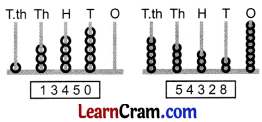# DAV Class 4 Maths Chapter 1 Worksheet 1 Solutions

The DAV Class 4 Maths Book Solutions and DAV Class 4 Maths Chapter 1 Worksheet 1 Solutions of Numbers up to 999999 offer comprehensive answers to textbook questions.

## DAV Class 4 Maths Ch 1 WS 1 Solutions

Question 1.
What number does the abacus show?351326103540000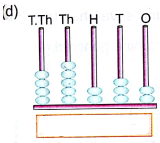45232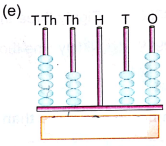6404644100

Question 2.
Represent the following numbers on the abacus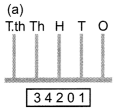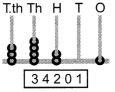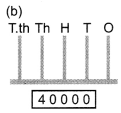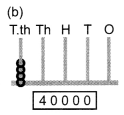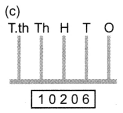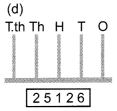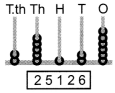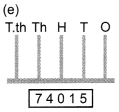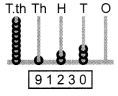### DAV Class 4 Maths Chapter 1 Worksheet 1 Notes

• When we add 1 to any greatest number of 1, 2, 3… digit number it becomes the smallest number of 2, 3, 4… digit number respectively.
For example, when we add 1 to the greatest 3-digit number 999 it becomes smallest 4-digit number 1000.
• A 6-digit number have the place value of lakh. The smallest 6-digit number is 100000 and the largest 6-digit number is 999999.
• There are six places of a 6-digit number i.e., Ones, Tens, Hundreds, Thousands and Lakhs.
• The place value of a digit in a numeral is the product of the digit and its place in the numeral.
• When we read numerals of a number, all the digits in same period are read together, and the name of period (except ones) is read along with them.
• When we write, we separate periods by leaving space or inserting commas between the periods.For example, 682934 is written as 6 82 934 or 6,82,934.
• The expanded form of a numeral is the sum of the place values of each digit of the numeral.
• While comparing digits as greater or smaller, we first compare digits in lakhs place, then in thousands place followed by hundreds, tens and ones place.

Number One More Than 9999:
9999 + 1 = 10000
9999 is the greatest 4 digit number.
10000 is the smallest 5 digit number.
The 5 places of a 5-digit number are

Ones, Tens, Hundreds, Thousands and Ten thousands.
On abacus, 10000 is shown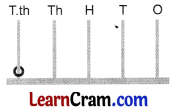10000 – Ten thousand

Some 5 – digit numbers